Ayush Gupta — October 7, 2021

This article was published as a part of the Data Science Blogathon

### Overview

Deep learning is the subfield of machine learning which is used to perform complex tasks such as speech recognition, text classification, etc. A deep learning model consists of activation function, input, output, hidden layers, loss function, etc. Any deep learning model tries to generalize the data using an algorithm and tries to make predictions on the unseen data. We need an algorithm that maps the examples of inputs to that of the outputs and an optimization algorithm. An optimization algorithm finds the value of the parameters(weights) that minimize the error when mapping inputs to outputs. These optimization algorithms or optimizers widely affect the accuracy of the deep learning model. They as well as affect the speed training of the model. But first of all the question arises what an optimizer really is?

While training the deep learning model, we need to modify each epoch’s weights and minimize the loss function. An optimizer is a function or an algorithm that modifies the attributes of the neural network, such as weights and learning rate. Thus, it helps in reducing the overall loss and improve the accuracy. The problem of choosing the right weights for the model is a daunting task, as a deep learning model generally consists of millions of parameters. It raises the need to choose a suitable optimization algorithm for your application. Hence understanding these algorithms is necessary before having a deep dive into the field.

You can use different optimizers to make changes in your weights and learning rate. However, choosing the best optimizer depends upon the application. As a beginner, one evil thought that comes to mind is that we try all the possibilities and choose the one that shows the best results. This might not be a problem initially, but when dealing with hundreds of gigabytes of data, even a single epoch can take a considerable amount of time. So randomly choosing an algorithm is no less than gambling with your precious time that you will realize sooner or later in your journey.

In this guide, we will learn about different optimizers used in building a deep learning model and the factors that could make you choose an optimizer instead of others for your application.

We are going to learn the following optimizers in this guide and look at the pros and cons of each algorithm. So that, at the end of the article, you will be able to compare various optimizers and the procedure they are based upon.

3. Stochastic Gradient descent with momentum
6. RMSProp

Before proceeding there are few terms that you should be familiar with.

Epoch – The number of times the algorithm runs on the whole training dataset.

Sample – A single row of a dataset.

Batch – It denotes the number of samples to be taken to for updating the model parameters.

Learning rate – It is a parameter that provides the model a scale of how much model weights should be updated.

Cost Function/Loss Function – A cost function is used to calculate the cost that is the difference between the predicted value and the actual value.

Weights/ Bias – The learnable parameters in a model that controls the signal between two neurons.

## Gradient Descent Deep Learning Optimizer

Gradient Descent can be considered as the popular kid among the class of optimizers. This optimization algorithm uses calculus to modify the values consistently and to achieve the local minimum. Before moving ahead, you might have the question of what a gradient is?

In simple terms, consider you are holding a ball resting at the top of a bowl. When you lose the ball, it goes along the steepest direction and eventually settles at the bottom of the bowl. A Gradient provides the ball in the steepest direction to reach the local minimum that is the bottom of the bowl.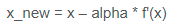The above equation means how the gradient is calculated. Here alpha is step size that represents how far to move against each gradient with each iteration.

1. It starts with some coefficients, sees their cost, and searches for cost value lesser than what it is now.
2. It moves towards the lower weight and updates the value of the coefficients.
3. The process repeats until the local minimum is reached. A local minimum is a point beyond which it can not proceed.

Gradient descent works best for most purposes. However, it has some downsides too. It is expensive to calculate the gradients if the size of the data is huge. Gradient descent works well for convex functions but it doesn’t know how far to travel along the gradient for nonconvex functions.

## Stochastic Gradient Descent Deep Learning Optimizer

At the end of the previous section, you learned why using gradient descent on massive data might not be the best option. To tackle the problem, we have stochastic gradient descent. The term stochastic means randomness on which the algorithm is based upon. In stochastic gradient descent, instead of taking the whole dataset for each iteration, we randomly select the batches of data. That means we only take few samples from the dataset.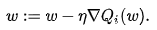The procedure is first to select the initial parameters w and learning rate n. Then randomly shuffle the data at each iteration to reach an approximate minimum.

Since we are not using the whole dataset but the batches of it for each iteration, the path took by the algorithm is full of noise as compared to the gradient descent algorithm. Thus, SGD uses a higher number of iterations to reach the local minima. Due to an increase in the number of iterations, the overall computation time increases. But even after increasing the number of iterations, the computation cost is still less than that of the gradient descent optimizer. So the conclusion is if the data is enormous and computational time is an essential factor, stochastic gradient descent should be preferred over batch gradient descent algorithm.

## Stochastic Gradient Descent with Momentum Deep Learning Optimizer

As discussed in the earlier section, you have learned that stochastic gradient descent takes a much more noisy path than the gradient descent algorithm. Due to this reason, it requires a more significant number of iterations to reach the optimal minimum and hence computation time is very slow. To overcome the problem, we use stochastic gradient descent with a momentum algorithm.

What the momentum does is helps in faster convergence of the loss function. Stochastic gradient descent oscillates between either direction of the gradient and updates the weights accordingly. However, adding a fraction of the previous update to the current update will make the process a bit faster. One thing that should be remembered while using this algorithm is that the learning rate should be decreased with a high momentum term.

In the above image, the left part shows the convergence graph of the stochastic gradient descent algorithm. At the same time, the right side shows SGD with momentum. From the image, you can compare the path chosen by both the algorithms and realize that using momentum helps reach convergence in less time. You might be thinking of using a large momentum and learning rate to make the process even faster. But remember that while increasing the momentum, the possibility of passing the optimal minimum also increases. This might result in poor accuracy and even more oscillations.

## Mini Batch Gradient Descent Deep Learning Optimizer

In this variant of gradient descent instead of taking all the training data, only a subset of the dataset is used for calculating the loss function. Since we are using a batch of data instead of taking the whole dataset, fewer iterations are needed. That is why the mini-batch gradient descent algorithm is faster than both stochastic gradient descent and batch gradient descent algorithms. This algorithm is more efficient and robust than the earlier variants of gradient descent. As the algorithm uses batching, all the training data need not be loaded in the memory, thus making the process more efficient to implement. Moreover, the cost function in mini-batch gradient descent is noisier than the batch gradient descent algorithm but smoother than that of the stochastic gradient descent algorithm. Because of this, mini-batch gradient descent is ideal and provides a good balance between speed and accuracy.

Despite, all that, the mini-batch gradient descent algorithm has some downsides too. It needs a hyperparameter that is “mini-batch-size”, which needs to be tuned to achieve the required accuracy. Although, the batch size of 32 is considered to be appropriate for almost every case. Also, in some cases, it results in poor final accuracy. Due to this, there needs a rise to look for other alternatives too.

The adaptive gradient descent algorithm is slightly different from other gradient descent algorithms. This is because it uses different learning rates for each iteration. The change in learning rate depends upon the difference in the parameters during training. The more the parameters get change, the more minor the learning rate changes. This modification is highly beneficial because real-world datasets contain sparse as well as dense features. So it is unfair to have the same value of learning rate for all the features. The Adagrad algorithm uses the below formula to update the weights. Here the alpha(t) denotes the different learning rates at each iteration, n is a constant, and E is a small positive to avoid division by 0.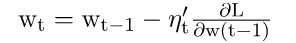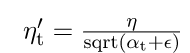The benefit of using Adagrad is that it abolishes the need to modify the learning rate manually. It is more reliable than gradient descent algorithms and their variants, and it reaches convergence at a higher speed.

One downside of AdaGrad optimizer is that it decreases the learning rate aggressively and monotonically. There might be a point when the learning rate becomes extremely small. This is because the squared gradients in the denominator keep accumulating, and thus the denominator part keeps on increasing. Due to small learning rates, the model eventually becomes unable to acquire more knowledge, and hence the accuracy of the model is compromised.

## RMS Prop(Root Mean Square) Deep Learning Optimizer

RMS prop is one of the popular optimizers among deep learning enthusiasts. This is maybe because it hasn’t been published but still very well know in the community. RMS prop is ideally an extension of the work RPPROP. RPPROP resolves the problem of varying gradients. The problem with the gradients is that some of them were small while others may be huge. So, defining a single learning rate might not be the best idea. RPPROP uses the sign of the gradient adapting the step size individually for each weight. In this algorithm, the two gradients are first compared for signs. If they have the same sign, we’re going in the right direction and hence increase the step size by a small fraction. Whereas, if they have opposite signs, we have to decrease the step size. Then we limit the step size, and now we can go for the weight update.

The problem with RPPROP is that it doesn’t work well with large datasets and when we want to perform mini-batch updates. So, achieving the robustness of RPPROP and efficiency of mini-batches at the same time was the main motivation behind the rise of RMS prop. RMS prop can also be considered an advancement in AdaGrad optimizer as it reduces the monotonically decreasing learning rate.

The algorithm mainly focuses on accelerating the optimization process by decreasing the number of function evaluations to reach the local minima. The algorithm keeps the moving average of squared gradients for every weight and divides the gradient by the square root of the mean square.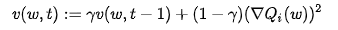where gamma is the forgetting factor. Weights are updated by the below formula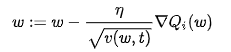In simpler terms, if there exists a parameter due to which the cost function oscillates a lot, we want to penalize the update of this parameter. Suppose you built a model to classify a variety of fishes. The model relies on the factor ‘color’ mainly to differentiate between the fishes. Due to which it makes a lot of errors. What RMS Prop does is, penalize the parameter ‘color’ so that it can rely on other features too. This prevents the algorithm from adapting too quickly to changes in the parameter ‘color’ compared to other parameters. This algorithm has several benefits as compared to earlier versions of gradient descent algorithms. The algorithm converges quickly and requires lesser tuning than gradient descent algorithms and their variants.

The problem with RMS Prop is that the learning rate has to be defined manually and the suggested value doesn’t work for every application.

AdaDelta can be seen as a more robust version of AdaGrad optimizer. It is based upon adaptive learning and is designed to deal with significant drawbacks of AdaGrad and RMS prop optimizer. The main problem with the above two optimizers is that the initial learning rate must be defined manually. One other problem is the decaying learning rate which becomes infinitesimally small at some point. Due to which a certain number of iterations later, the model can no longer learn new knowledge.

To deal with these problems, AdaDelta uses two state variables to store the leaky average of the second moment gradient and a leaky average of the second moment of change of parameters in the model.

Here St and delta Xt denotes the state variables, g’t denotes rescaled gradient, delta Xt-1 denotes squares rescaled gradients, and epsilon represents a small positive integer to handle division by 0.

The name adam is derived from adaptive moment estimation. This optimization algorithm is a further extension of stochastic gradient descent to update network weights during training. Unlike maintaining a single learning rate through training in SGD, Adam optimizer updates the learning rate for each network weight individually. The creators of the Adam optimization algorithm know the benefits of AdaGrad and RMSProp algorithms, which are also extensions of the stochastic gradient descent algorithms. Hence the Adam optimizers inherit the features of both Adagrad and RMS prop algorithms. In adam, instead of adapting learning rates based upon the first moment(mean) as in RMS Prop, it also uses the second moment of the gradients. We mean the uncentred variance by the second moment of the gradients(we don’t subtract the mean).

The adam optimizer has several benefits, due to which it is used widely. It is adapted as a benchmark for deep learning papers and recommended as a default optimization algorithm. Moreover, the algorithm is straightforward to implement, has faster running time, low memory requirements, and requires less tuning than any other optimization algorithm.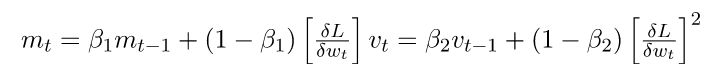The above formula represents the working of adam optimizer. Here B1 and B2 represent the decay rate of the average of the gradients.

If the adam optimizer uses the good properties of all the algorithms and is the best available optimizer, then why shouldn’t you use Adam in every application? And what was the need to learn about other algorithms in depth? This is because even Adam has some downsides. It tends to focus on faster computation time, whereas algorithms like stochastic gradient descent focus on data points. That’s why algorithms like SGD generalize the data in a better manner at the cost of low computation speed. So, the optimization algorithms can be picked accordingly depending upon the requirements and the type of data.

The above visualizations create a better picture in mind and help in comparing the results of various optimization algorithms.

## Hands-on Optimizers

We have learned enough theory and now we need to do some practical analysis. It’s time to try what we have learned and compare the results by choosing different optimizers on a simple neural network. As we are talking of keeping things simple, what’s better than the MNIST dataset. We will train a simple model using some basic layers, keeping the batch size, and epochs the same but with different optimizers.  For the sake of fairness, we will use the default values with each optimizer.

The steps for building the network are given below.

Import Libraries

```import keras
from keras.datasets import mnist
from keras.models import Sequential
from keras.layers import Dense, Dropout, Flatten
from keras.layers import Conv2D, MaxPooling2D
from keras import backend as K
(x_train, y_train), (x_test, y_test) = mnist.load_data()
print(x_train.shape, y_train.shape)```

```x_train= x_train.reshape(x_train.shape,28,28,1)
x_test=  x_test.reshape(x_test.shape,28,28,1)
input_shape=(28,28,1)
y_train=keras.utils.to_categorical(y_train)#,num_classes=)
y_test=keras.utils.to_categorical(y_test)#, num_classes)
x_train= x_train.astype('float32')
x_test= x_test.astype('float32')
x_train /= 255
x_test /=255```

Build Model

```batch_size=64

num_classes=10

epochs=10

def build_model(optimizer):

model=Sequential()

model.compile(loss=keras.losses.categorical_crossentropy, optimizer= optimizer, metrics=['accuracy'])

return model```

Train Model

```optimizers = ['Adadelta', 'Adagrad', 'Adam', 'RMSprop', 'SGD']

for i in optimizers:

model = build_model(i)

hist=model.fit(x_train, y_train, batch_size=batch_size, epochs=epochs, verbose=1, validation_data=(x_test,y_test))```

We have run our model with a batch size of 64 for 10 epochs. After trying the different optimizers, the results we get are pretty interesting. Before analyzing the results, what do you think, will be the best optimizer for this dataset?

 Optimizer Epoch 1 Val accuracy | Val loss Epoch 5 Val accuracy | Val loss Epoch 10 Val accuracy | Val loss Total Time Adadelta .4612 | 2.2474 .7776 | 1.6943 .8375 | 0.9026 8:02 min Adagrad .8411 | .7804 .9133 | .3194 .9286 | 0.2519 7:33 min Adam .9772 | .0701 .9884 | .0344 .9908 | .0297 7:20 min RMSprop .9783 | .0712 .9846 | .0484 .9857 | .0501 10:01 min SGD with momentum .9168 | .2929 .9585 | .1421 .9697 | .1008 7:04 min SGD .9124 | .3157 .9569 | 1451 .9693 | .1040 6:42 min

The above table shows the validation accuracy and loss at different epochs. It also contains the total time that the model took to run on 10 epochs for each optimizer. From the above table, we can make the following analysis

• The adam optimizer shows the best accuracy in a satisfactory amount of time.
• RMSprop shows similar accuracy to that of Adam but with comparatively much larger computation time.
• Surprisingly, the SGD algorithm took the least time to train and produces good results as well. But to reach the accuracy of the Adam optimizer, SGD will require more iterations and hence the computation time will increase.
•  SGD with momentum shows similar accuracy to SGD with unexpectedly larger computation time. This means the value of momentum taken needs to be optimized.

Please let me know if you think I am missing something.

You can analyze the accuracy of each optimizer with each epoch from the below graph

## Conclusion

This article taught us how an optimization algorithm can affect the deep learning model in terms of accuracy, speed, and efficiency. We learned about various algorithms, and hopefully, you were able to compare the algorithms from one another. You also learned when to use which algorithm and what could be the downsides of using that algorithm. To refresh your memory we will go through a summary of every optimization algorithm that we have covered in this guide.

SGD is a very basic algorithm and is hardly used in applications now due to its slow computation speed. One more problem with that algorithm is the constant learning rate for every epoch. Moreover, it is not able to handle saddle points very well. Adagrad works better than stochastic gradient descent generally due to frequent updates in the learning rate. It is best when used for dealing with sparse data. RMSProp shows similar results to that of gradient descent algorithm with momentum, just differs the way by which the gradients are calculated. Lastly comes the Adam optimizer that inherits the good features of RMSProp and other algorithms. The results of the Adam optimizer are generally better than every other optimization algorithms, have faster computation time, and require fewer parameters for tuning. Because of all that, Adam is recommended as the default optimizer for most of the applications. Choosing the Adam optimizer for your application might give you the best probability of getting the best results.

At last, we learned that even Adam optimizer has some downsides. Also, there are cases when algorithms like SGD might be beneficial and perform better than Adam optimizer. So, it is of utmost importance to know your requirements and the type of data you are dealing with to choose the best optimization algorithm and to achieve outstanding results.